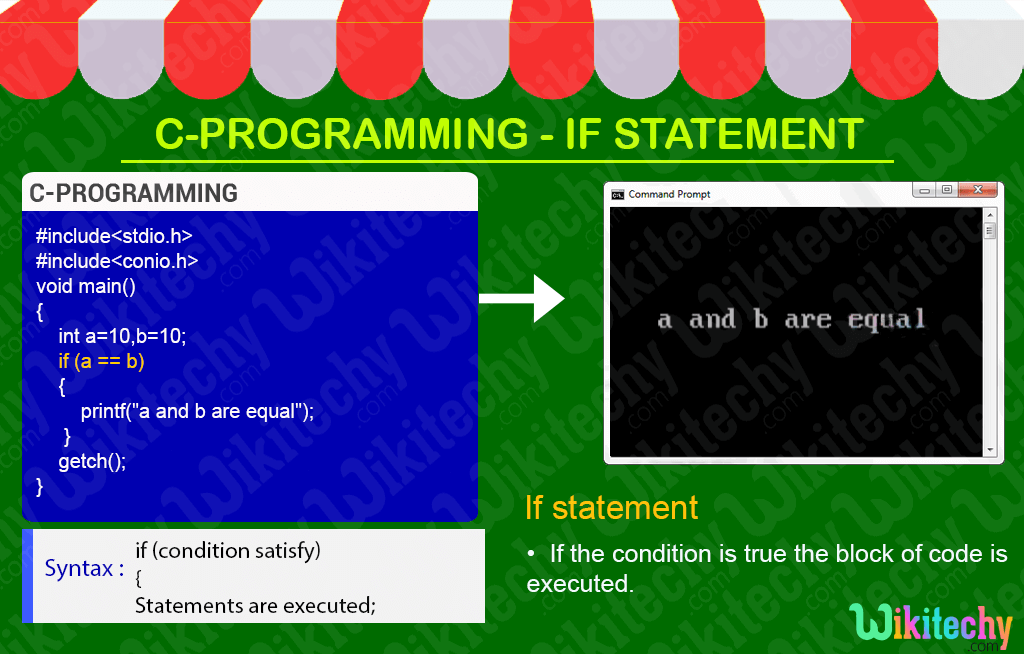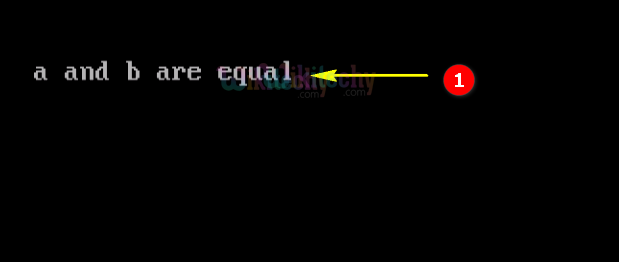# C - If StatementLearn C - C tutorial - C if statement - C examples - C programs

## C If Statement - Definition and Usage

• In C- Programming the if statement is a conditional branching statement that is if the condition is satisfied the respective block of code is executed.## C Code - Explanation1. Here we declare the variable a &b as “10” with its data type as integer.
2. In this statement we check the value for the variable a&b which are equal or not, because if the condition is true the printf statement prints “a” and “b” are equal”..

## Output :1. Here the value of the variable “a & b” are equal so “if” condition will be satisfied and the output print statement will be displayed as “a and b are equal”.